+
Solving Multi-step Equations
Solving Linear Equations and Inequalities
0
of 0 possible points

# Solving Multi-step Equations

Author: Sophia Tutorial
##### Description:

Solve multi-step equations involving roots.

(more)

Sophia’s self-paced online courses are a great way to save time and money as you earn credits eligible for transfer to many different colleges and universities.*

No credit card required

46 Sophia partners guarantee credit transfer.

299 Institutions have accepted or given pre-approval for credit transfer.

* The American Council on Education's College Credit Recommendation Service (ACE Credit®) has evaluated and recommended college credit for 33 of Sophia’s online courses. Many different colleges and universities consider ACE CREDIT recommendations in determining the applicability to their course and degree programs.

Tutorial
what's covered
1. Solving an Equation
2. Multi-step Equations with Distribution
3. A Variable in the Denominator
4. Equations with a Root

# 1. Solving an Equation

When solving an equation, our main goal is to isolate the variable we wish to solve for onto one side of the equation, and then evaluate the expression on the other side of the equation. To do this, we use inverse operations to undo the operations on our variable. With single step equations, only one operation is applied to our variable, so only one inverse operation must be applied. Let's review these inverse operations.

Operation Inverse Operation
Multiplication Division
Division Multiplication
Powers Roots
Roots Powers

What do we do when we have several operations being performed on the variable we wish to solve for? We know that we must perform inverse operations to both sides of the equation (due to the Rule of Equality for equations), but in what order? Think about the order of operations. These operations are applied to the variable following the order of operations. To undo this, we apply inverse operations in the reverse order of operations

big idea
To solve multi-step equations, apply inverse operations to both sides of the equation, following the reverse order of operations.

# 2. An Equation with Distribution

Let's take a look at an example of an equation involving distribution. Generally, there are two options. Option 1 is to divide by the outside factor, eliminating the need to distribute anything. Option 2 is to simplify the expression by distributing the factor. We will see how to solve an equation both ways: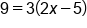Divide the equation by 3Undo -5 by adding 5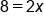Undo x2 by dividing by 2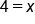Our SolutionDistribute 3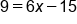Undo -15 by adding 15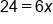Undo x6 by dividing by 6Our Solution

# 3. A Variable in the Denominator

When a variable appears in the denominator of a fraction, it can be difficult to isolate that variable until it is moved into a numerator. When the denominator contains the variable, the first step we take is to multiply the entire equation by the expression in the denominator. This eliminates the variable from the denominator on one side of the equation, and makes it part of the numerator on the other side of the equation. This is illustrated in the example below: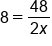Multiply the equation by 2x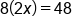Distribute 8 into 2x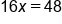Divide by 16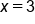Our Solution

hint
Notice that when clearing the variable in the denominator, we multiply the equation by the entire denominator, not just by the variable alone.

# 4. An Equation involving a Power or a Root

So far we have seen how to apply inverse operations to undo addition, subtraction, multiplication and division. Lastly, let's see how to apply power and roots to solve a multi-step equation.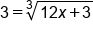Undo the cube root by cubing both sides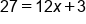Undo +3 by subtracting 3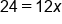Undo x12 by dividing 12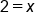Our Solution

As we can see with the example above, we had an expression underneath a cube root. To undo this, we cubed both sides of the equation. We were left with the expression alone, without a radical, and 3 cubed on the other side. From there, we were able to solve for x by applying our familiar inverse operations.

summary
The process for solving an equation involves using inverse operations in the reverse order of operations or PEMDAS backwards. If possible, it is best to simplify the equation before using those inverse operations. If a variable is in the denominator of a fraction, you need to multiply both sides of the equation by that variable to solve the equation. Finally, if solving an equation with a power or root, you need to isolate the radical and use a power to cancel it out before doing anything underneath the radical.

Rating